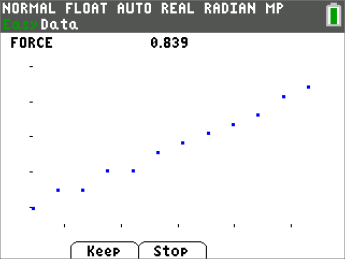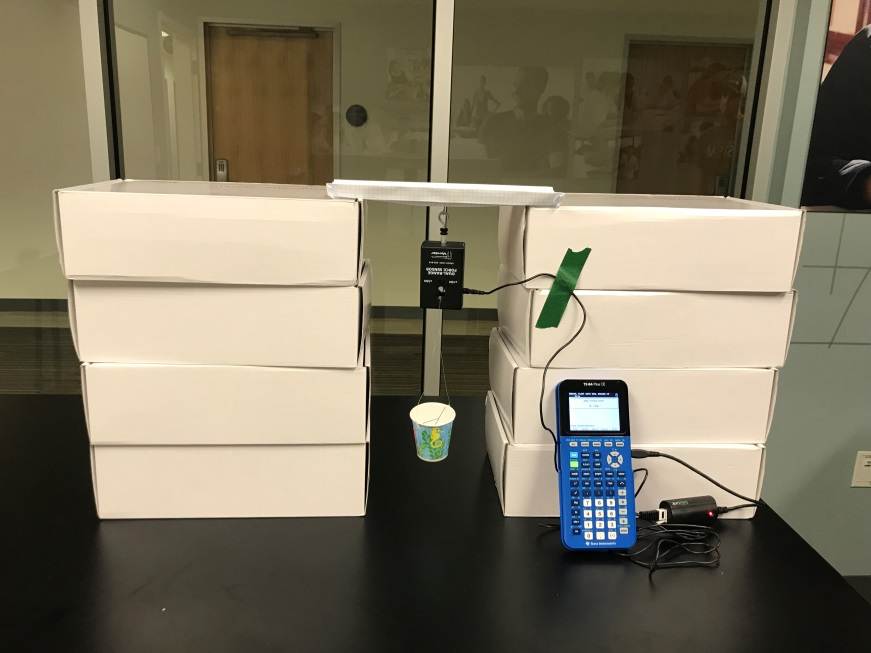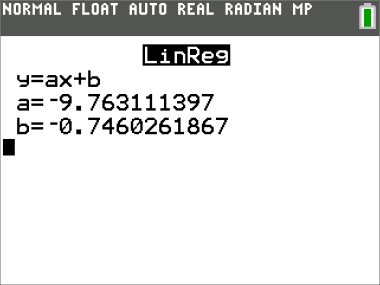Activities

•• Subject Area

• Math: Algebra I: Data Analysis

• Author9-12

45 Minutes

• Device
•TI-84 Plus CE
• TI-84 Plus
•TI-84 Plus C Silver Edition
• Accessories

Sensor - Dual Range Force

• Report an Issue

Building Bridges Between Engineering and MathematicsActivity Overview

In this activity, students walk through an engineering design project that has a cool algebra twist. Students will develop a foundational relationship between mass, force, and gravity.

Key Steps

•Students are challenged to build a bridge strong enough to support the force sensor and some washers using only a single sheet of paper.

•Students collect force data using the Vernier Dual Range Force sensor, washers, and their graphing calculator.

•Students analyze the data by making a scatterplot, fitting a linear regression curve to the data, then interpreting the slope and intercept in context.